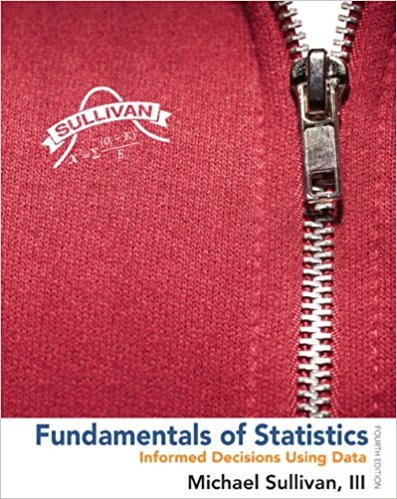### Solution Found!# Solved: The Empirical Rule The following data represent

Chapter 9, Problem 23

#### (choose chapter or problem)

Get Unlimited Answers! Check out our subscriptions

QUESTION:

The Empirical Rule The following data represent the weights (in grams) of a random sample of 50 M&M plain candies.

 0.87 0.88 0.82 0.9 0.9 0.84 0.84 0.91 0.94 0.86 0.86 0.86 0.88 0.87 0.89 0.91 0.86 0.87 0.93 0.88 0.83 0.95 0.87 0.93 0.91 0.85 0.91 0.91 0.86 0.89 0.87 0.84 0.88 0.88 0.89 0.79 0.82 0.83 0.9 0.88 0.84 0.93 0.81 0.9 0.88 0.92 0.85 0.84 0.84 0.86

Source: Michael Sullivan

(a) Determine the sample standard deviation weight. Express your answer rounded to three decimal places.

(b) On the basis of the histogram drawn in Section 3.1, Problem 27, comment on the appropriateness of using the Empirical Rule to make any general statements about the weights of M&Ms.

(c) Use the Empirical Rule to determine the percentage of M&Ms with weights between 0.803 and 0.947 gram. Hint: $$\bar{x}$$ = 0.875

(d) Determine the actual percentage of M&Ms that weigh between 0.803 and 0.947 gram, inclusive.

(e) Use the Empirical Rule to determine the percentage of M&Ms with weights more than 0.911 gram.

(f) Determine the actual percentage of M&Ms that weigh more than 0.911 gram.

Step 1 of 2

a) First we need to find mean.

$$\begin{array}{l} \bar{x}=\frac{\sum_{i=1}^{n} x_{i}}{n} \\ \bar{x}=\frac{38.3083}{50} \\ \bar{x}=0.766 \end{array}$$

Here standard deviation is

$$\begin{array}{l} s^{2}=\frac{\Sigma\left(x_{i}-\bar{x}\right)^{2}}{n-1}\\ s^{2}=0.0012 \end{array}$$

Then the sample variance is

$$\begin{array}{l} S=\sqrt{\frac{\Sigma\left(x_{i}-\bar{x}\right)^{2}}{n-1}} \\ S=\sqrt{0.0012} \\ S=0.036 \end{array}$$

Hence the sample variance is s = 0.036 gram.

Become a subscriber and get unlimited answers!